Polar Coordinates

We have the following relationships between polar coordinates and rectangular coordinates: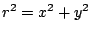Ex 1 Find a set of polar coordinates for the point with rectangular coordinates.

Sol, so we can take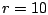. Since, we can take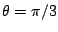. Therefore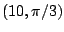is a set of polar coordinates for this point.

Ex 2 Find rectangular coordinates for the point which has polar coordinates.

Sol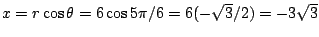and, so the point has rectangular coordinates.

Pr A Find a polar equation for the circle with rectangular equation.

Pr B Find the standard form of the rectangular equation of the circle with polar equation.

Pr C Find two sets of polar coordinates for the point with rectangular coordinates.

Pr 1 Find a set of polar coordinates for the point with rectangular coordinates.

Pr 2 Find a set of polar coordinates for the point with rectangular coordinates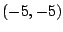.

Pr 3 Find rectangular coordinates for the point which has polar coordinates.

Pr 4 Find a polar equation for the line with equationin rectangular coordinates.

Pr 5 Find a polar equation for the line with equationin rectangular coordinates.

Pr 6 Find a polar equation for the circle with equationin rectangular coordinates.

Pr 7 Find a rectangular equation for the cardioid with polar equation.

Pr 8 Find the points of intersection of the curves with polar equations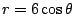and.